# Build an Emotion-Based Dog Filter in Python 3 using Computer VisionIn this tutorial, you built a face detector and dog filter in Python 3 using Computer Vision and employed Machine Learning models to apply masks based on detected emotions.

In this tutorial, you built a face detector and dog filter in Python 3 using Computer Vision and employed Machine Learning models to apply masks based on detected emotions.

### Introduction

Computer vision is a subfield of computer science that aims to extract a higher-order understanding from images and videos. This field includes tasks such as object detection, image restoration (matrix completion), and optical flow. Computer vision powers technologies such as self-driving car prototypes, employee-less grocery stores, fun Snapchat filters, and your mobile device's face authenticator.

In this tutorial, you will explore computer vision as you use pre-trained models to build a Snapchat-esque dog filter. For those unfamiliar with Snapchat, this filter will detect your face and then superimpose a dog mask on it. You will then train a face-emotion classifier so that the filter can pick dog masks based on emotion, such as a corgi for happy or a pug for sad. Along the way, you will also explore related concepts in both ordinary least squares and computer vision, which will expose you to the fundamentals of machine learning.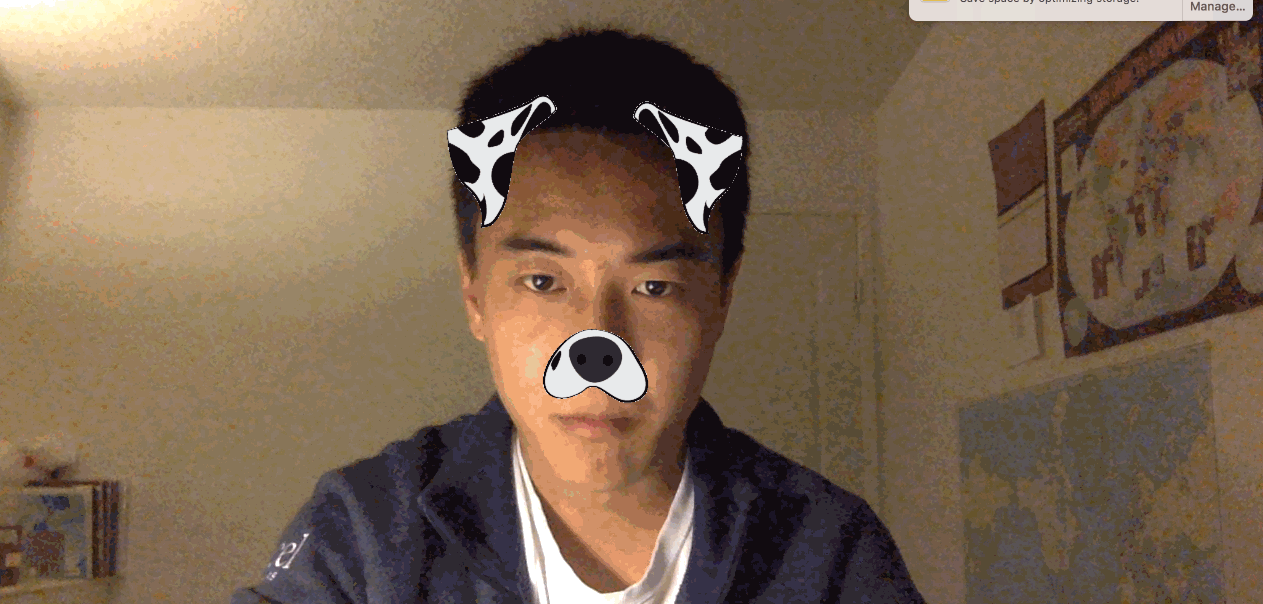As you work through the tutorial, you'll use `OpenCV`, a computer-vision library, `numpy` for linear algebra utilities, and `matplotlib` for plotting. You'll also apply the following concepts as you build a computer-vision application:

• Ordinary least squares as a regression and classification technique.
• The basics of stochastic gradient neural networks.

While not necessary to complete this tutorial, you'll find it easier to understand some of the more detailed explanations if you're familiar with these mathematical concepts:

• Fundamental linear algebra concepts: scalars, vectors, and matrices.
• Fundamental calculus: how to take a derivative.

You can find the complete code for this tutorial at https://github.com/do-community/emotion-based-dog-filter.

Let's get started.

## Prerequisites

To complete this tutorial, you will need the following:

## Step 1 — Creating The Project and Installing Dependencies

Let's create a workspace for this project and install the dependencies we'll need. We'll call our workspace `DogFilter`:

``````mkdir ~/DogFilter
``````

Navigate to the `DogFilter` directory:

``````cd ~/DogFilter
``````

Then create a new Python virtual environment for the project:

``````python3 -m venv dogfilter
``````

``````source dogfilter/bin/activate
``````

The prompt changes, indicating the environment is active. Now install PyTorch, a deep-learning framework for Python that we'll use in this tutorial. The installation process depends on which operating system you're using.

On macOS, install Pytorch with the following command:

``````python -m pip install torch==0.4.1 torchvision==0.2.1
``````

On Linux, use the following commands:

``````pip install http://download.pytorch.org/whl/cpu/torch-0.4.1-cp35-cp35m-linux_x86_64.whl
pip install torchvision
``````

And for Windows, install Pytorch with these commands:

``````pip install http://download.pytorch.org/whl/cpu/torch-0.4.1-cp35-cp35m-win_amd64.whl
pip install torchvision
``````

Now install prepackaged binaries for `OpenCV` and `numpy`, which are computer vision and linear algebra libraries, respectively. The former offers utilities such as image rotations, and the latter offers linear algebra utilities such as a matrix inversion.

``````python -m pip install opencv-python==3.4.3.18 numpy==1.14.5
``````

Finally, create a directory for our assets, which will hold the images we'll use in this tutorial:

``````mkdir assets
``````

With the dependencies installed, let's build the first version of our filter: a face detector.

## Step 2 — Building a Face Detector

Our first objective is to detect all faces in an image. We'll create a script that accepts a single image and outputs an annotated image with the faces outlined with boxes.

Fortunately, instead of writing our own face detection logic, we can use pre-trained models. We'll set up a model and then load pre-trained parameters. OpenCV makes this easy by providing both.

OpenCV provides the model parameters in its source code. but we need the absolute path to our locally-installed OpenCV to use these parameters. Since that absolute path may vary, we'll download our own copy instead and place it in the `assets` folder:

``````wget -O assets/haarcascade_frontalface_default.xml https://github.com/opencv/opencv/raw/master/data/haarcascades/haarcascade_frontalface_default.xml
``````

The `-O` option specifies the destination as `assets/haarcascade_frontalface_default.xml`. The second argument is the source URL.

We'll detect all faces in the following image from Pexels (CC0, link to original image).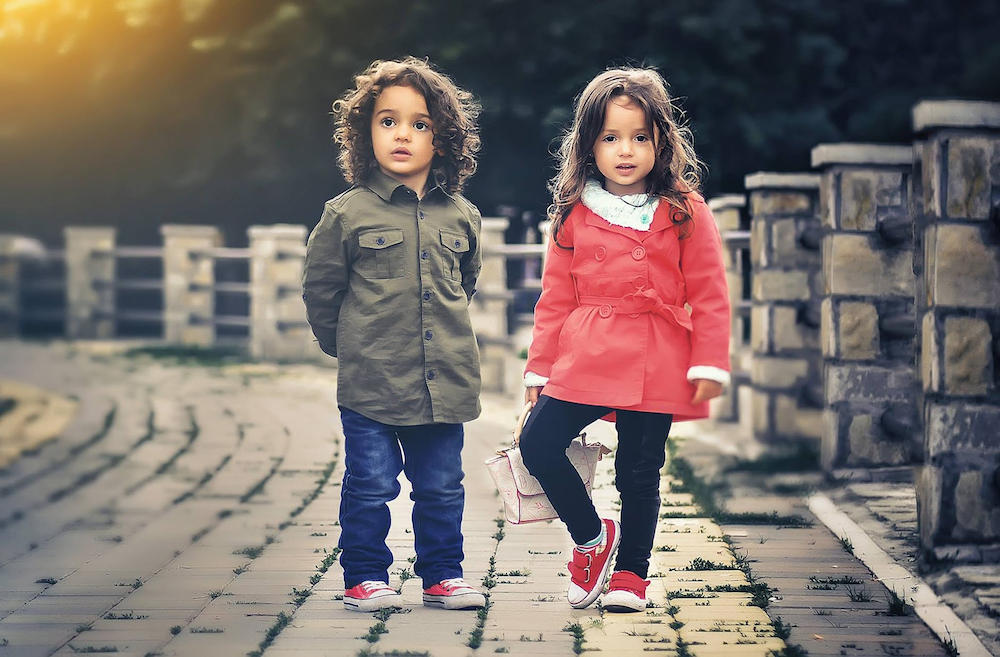First, download the image. The following command saves the downloaded image as `children.png` in the `assets` folder:

``````wget -O assets/children.png https://assets.digitalocean.com/articles/python3_dogfilter/CfoBWbF.png
``````

To check that the detection algorithm works, we will run it on an individual image and save the resulting annotated image to disk. Create an `outputs` folder for these annotated results.

``````mkdir outputs
``````

Now create a Python script for the face detector. Create the file `step_1_face_detect` using `nano` or your favorite text editor:

``````nano step_2_face_detect.py
``````

Add the following code to the file. This code imports OpenCV, which contains the image utilities and face classifier. The rest of the code is typical Python program boilerplate.

step_2_face_detect.py

``````"""Test for face detection"""

import cv2

def main():
pass

if __name__ == '__main__':
main()
``````

Now replace `pass` in the `main` function with this code which initializes a face classifier using the OpenCV parameters you downloaded to your `assets` folder:

step_2_face_detect.py

``````def main():
# initialize front face classifier
``````

Next, add this line to load the image `children.png`.

step_2_face_detect.py

``````    frame = cv2.imread('assets/children.png')
``````

Then add this code to convert the image to black and white, as the classifier was trained on black-and-white images. To accomplish this, we convert to grayscale and then discretize the histogram:

step_2_face_detect.py

``````    # Convert to black-and-white
gray = cv2.cvtColor(frame, cv2.COLOR_BGR2GRAY)
blackwhite = cv2.equalizeHist(gray)
``````

Then use OpenCV's `[detectMultiScale](https://docs.opencv.org/2.4/modules/objdetect/doc/cascade_classification.html#cascadeclassifier-detectmultiscale)` function to detect all faces in the image.

step_2_face_detect.py

``````    rects = cascade.detectMultiScale(
blackwhite, scaleFactor=1.3, minNeighbors=4, minSize=(30, 30),
``````
• `scaleFactor` specifies how much the image is reduced along each dimension.
• `minNeighbors` denotes how many neighboring rectangles a candidate rectangle needs to be retained.
• `minSize` is the minimum allowable detected object size. Objects smaller than this are discarded.

The return type is a list of tuples, where each tuple has four numbers denoting the minimum x, minimum y, width, and height of the rectangle in that order.

Iterate over all detected objects and draw them on the image in green using `[cv2.rectangle](https://docs.opencv.org/2.4/modules/core/doc/drawing_functions.html#rectangle)`:

step_2_face_detect.py

``````    for x, y, w, h in rects:
cv2.rectangle(frame, (x, y), (x + w, y + h), (0, 255, 0), 2)
``````
• The second and third arguments are opposing corners of the rectangle.
• The fourth argument is the color to use. `(0, 255, 0)` corresponds to green for our RGB color space.
• The last argument denotes the width of our line.

Finally, write the image with bounding boxes into a new file at `outputs/children_detected.png`:

step_2_face_detect.py

``````    cv2.imwrite('outputs/children_detected.png', frame)
``````

Your completed script should look like this:

step_2_face_detect.py

``````"""Tests face detection for a static image."""

import cv2

def main():

# initialize front face classifier

# Convert to black-and-white
gray = cv2.cvtColor(frame, cv2.COLOR_BGR2GRAY)
blackwhite = cv2.equalizeHist(gray)

blackwhite, scaleFactor=1.3, minNeighbors=4, minSize=(30, 30),

for x, y, w, h in rects:
cv2.rectangle(frame, (x, y), (x + w, y + h), (0, 255, 0), 2)

cv2.imwrite('outputs/children_detected.png', frame)

if __name__ == '__main__':
main()
``````

Save the file and exit your editor. Then run the script:

``````python step_2_face_detect.py
``````

Open `outputs/children_detected.png`. You'll see the following image that shows the faces outlined with boxes: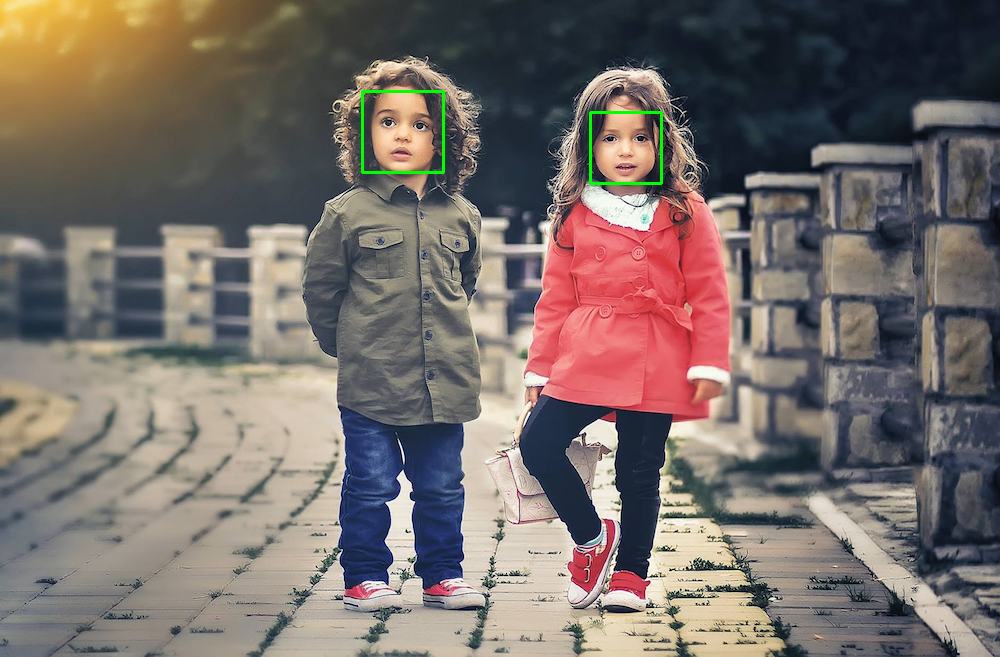At this point, you have a working face detector. It accepts an image as input and draws bounding boxes around all faces in the image, outputting the annotated image. Now let's apply this same detection to a live camera feed.

## Step 3 — Linking the Camera Feed

The next objective is to link the computer's camera to the face detector. Instead of detecting faces in a static image, you'll detect all faces from your computer's camera. You will collect camera input, detect and annotate all faces, and then display the annotated image back to the user. You'll continue from the script in Step 2, so start by duplicating that script:

``````cp step_2_face_detect.py step_3_camera_face_detect.py
``````

Then open the new script in your editor:

``````nano step_3_camera_face_detect.py
``````

You will update the `main` function by using some elements from this test script from the official OpenCV documentation. Start by initializing a `VideoCapture` object that is set to capture live feed from your computer's camera. Place this at the start of the `main` function, before the other code in the function:

step_3_camera_face_detect.py

``````def main():
cap = cv2.VideoCapture(0)
...
``````

Starting from the line defining `frame`, indent all of your existing code, placing all of the code in a `while` loop.

step_3_camera_face_detect.py

``````    while True:
...
for x, y, w, h in rects:
cv2.rectangle(frame, (x, y), (x + w, y + h), (0, 255, 0), 2)

cv2.imwrite('outputs/children_detected.png', frame)
``````

Replace the line defining `frame` at the start of the `while` loop. Instead of reading from an image on disk, you're now reading from the camera:

step_3_camera_face_detect.py

``````    while True:
# frame = cv2.imread('assets/children.png') # DELETE ME
# Capture frame-by-frame
``````

Replace the line `cv2.imwrite(...)` at the end of the `while` loop. Instead of writing an image to disk, you'll display the annotated image back to the user's screen:

step_3_camera_face_detect.py

``````      cv2.imwrite('outputs/children_detected.png', frame)  # DELETE ME
# Display the resulting frame
cv2.imshow('frame', frame)
``````

Also, add some code to watch for keyboard input so you can stop the program. Check if the user hits the `q` character and, if so, quit the application. Right after `cv2.imshow(...)` add the following:

step_3_camera_face_detect.py

``````...
cv2.imshow('frame', frame)
if cv2.waitKey(1) & 0xFF == ord('q'):
break
...
``````

The line `cv2.waitkey(1)` halts the program for 1 millisecond so that the captured image can be displayed back to the user.

Finally, release the capture and close all windows. Place this outside of the `while` loop to end the `main` function.

step_3_camera_face_detect.py

``````...

while True:
...

cap.release()
cv2.destroyAllWindows()
``````

Your script should look like the following:

step_3_camera_face_detect.py

``````"""Test for face detection on video camera.

Move your face around and a green box will identify your face.
With the test frame in focus, hit `q` to exit.
Note that typing `q` into your terminal will do nothing.
"""

import cv2

def main():
cap = cv2.VideoCapture(0)

# initialize front face classifier

while True:
# Capture frame-by-frame

# Convert to black-and-white
gray = cv2.cvtColor(frame, cv2.COLOR_BGR2GRAY)
blackwhite = cv2.equalizeHist(gray)

# Detect faces
blackwhite, scaleFactor=1.3, minNeighbors=4, minSize=(30, 30),

# Add all bounding boxes to the image
for x, y, w, h in rects:
cv2.rectangle(frame, (x, y), (x + w, y + h), (0, 255, 0), 2)

# Display the resulting frame
cv2.imshow('frame', frame)
if cv2.waitKey(1) & 0xFF == ord('q'):
break

# When everything done, release the capture
cap.release()
cv2.destroyAllWindows()

if __name__ == '__main__':
main()
``````

Save the file and exit your editor.

Now run the test script.

``````python step_3_camera_face_detect.py
``````

This activates your camera and opens a window displaying your camera's feed. Your face will be boxed by a green square in real time: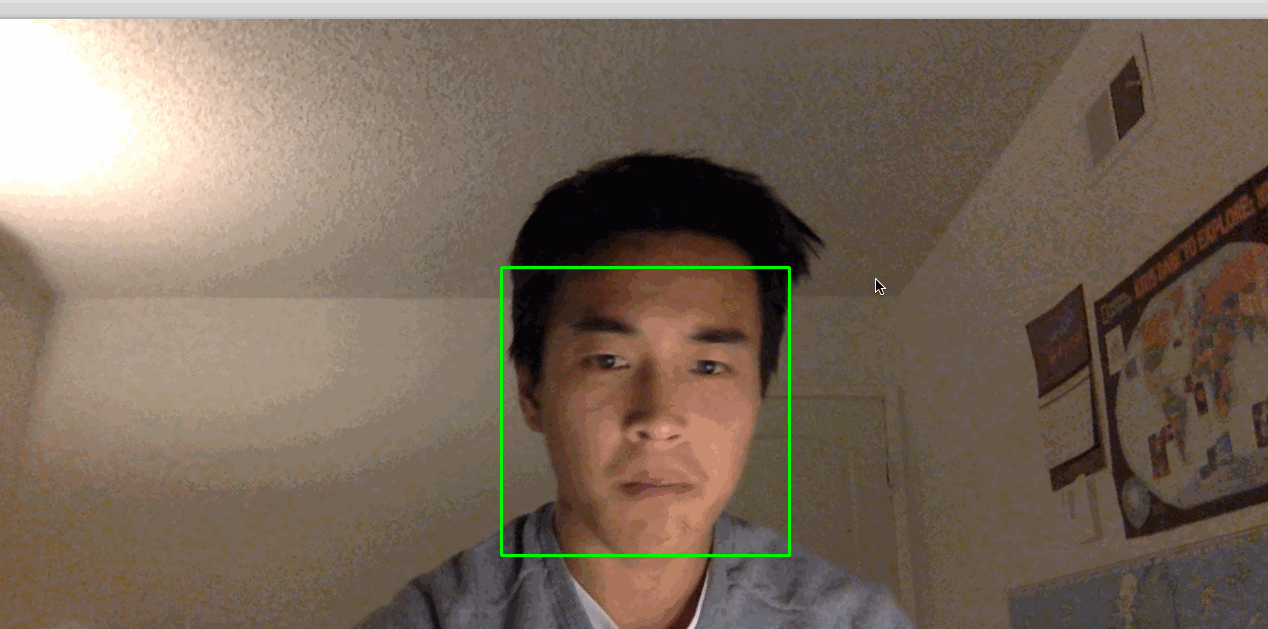Note: If you find that you have to hold very still for things to work, the lighting in the room may not be adequate. Try moving to a brightly lit room where you and your background have high constrast. Also, avoid bright lights near your head. For example, if you have your back to the sun, this process might not work very well.

Our next objective is to take the detected faces and superimpose dog masks on each one.

## Step 4 — Building the Dog Filter

Before we build the filter itself, let's explore how images are represented numerically. This will give you the background needed to modify images and ultimately apply a dog filter.

Let's look at an example. We can construct a black-and-white image using numbers, where `0` corresponds to black and `1` corresponds to white.

Focus on the dividing line between 1s and 0s. What shape do you see?

``````0 0 0 0 0 0 0 0 0
0 0 0 0 1 0 0 0 0
0 0 0 1 1 1 0 0 0
0 0 1 1 1 1 1 0 0
0 0 0 1 1 1 0 0 0
0 0 0 0 1 0 0 0 0
0 0 0 0 0 0 0 0 0
``````

The image is a diamond. If save this matrix of values as an image. This gives us the following picture: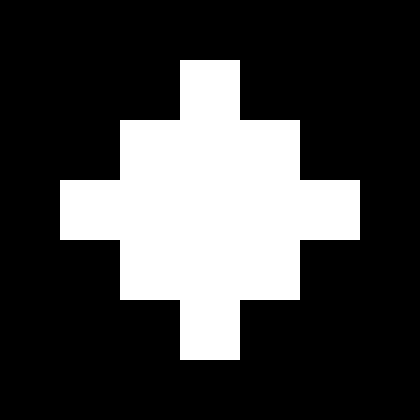We can use any value between 0 and 1, such as 0.1, 0.26, or 0.74391. Numbers closer to 0 are darker and numbers closer to 1 are lighter. This allows us to represent white, black, and any shade of gray. This is great news for us because we can now construct any grayscale image using 0, 1, and any value in between. Consider the following, for example. Can you tell what it is? Again, each number corresponds to the color of a pixel.

``````1  1  1  1  1  1  1  1  1  1  1  1
1  1  1  1  0  0  0  0  1  1  1  1
1  1  0  0 .4 .4 .4 .4  0  0  1  1
1  0 .4 .4 .5 .4 .4 .4 .4 .4  0  1
1  0 .4 .5 .5 .5 .4 .4 .4 .4  0  1
0 .4 .4 .4 .5 .4 .4 .4 .4 .4 .4  0
0 .4 .4 .4 .4  0  0 .4 .4 .4 .4  0
0  0 .4 .4  0  1 .7  0 .4 .4  0  0
0  1  0  0  0 .7 .7  0  0  0  1  0
1  0  1  1  1  0  0 .7 .7 .4  0  1
1  0 .7  1  1  1 .7 .7 .7 .7  0  1
1  1  0  0 .7 .7 .7 .7  0  0  1  1
1  1  1  1  0  0  0  0  1  1  1  1
1  1  1  1  1  1  1  1  1  1  1  1
``````

Re-rendered as an image, you can now tell that this is, in fact, a Poké Ball: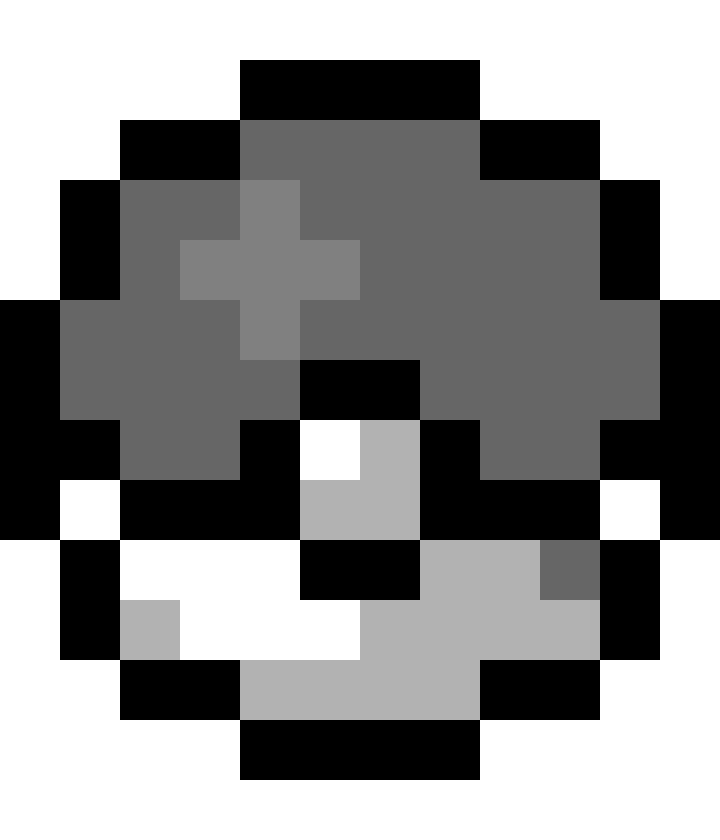You've now seen how black-and-white and grayscale images are represented numerically. To introduce color, we need a way to encode more information. An image has its height and width expressed as `h x w`.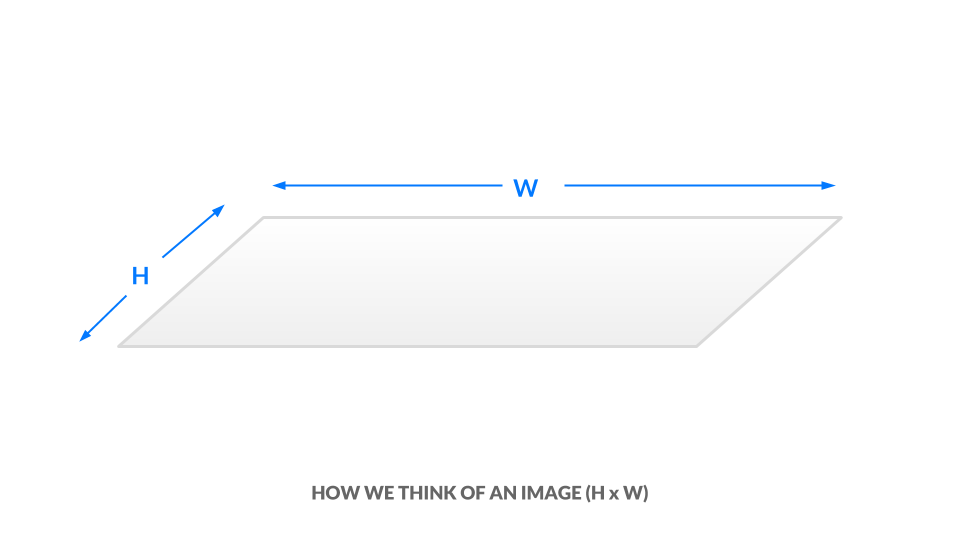In the current grayscale representation, each pixel is one value between 0 and 1. We can equivalently say our image has dimensions `h x w x 1`. In other words, every `(x, y)` position in our image has just one value.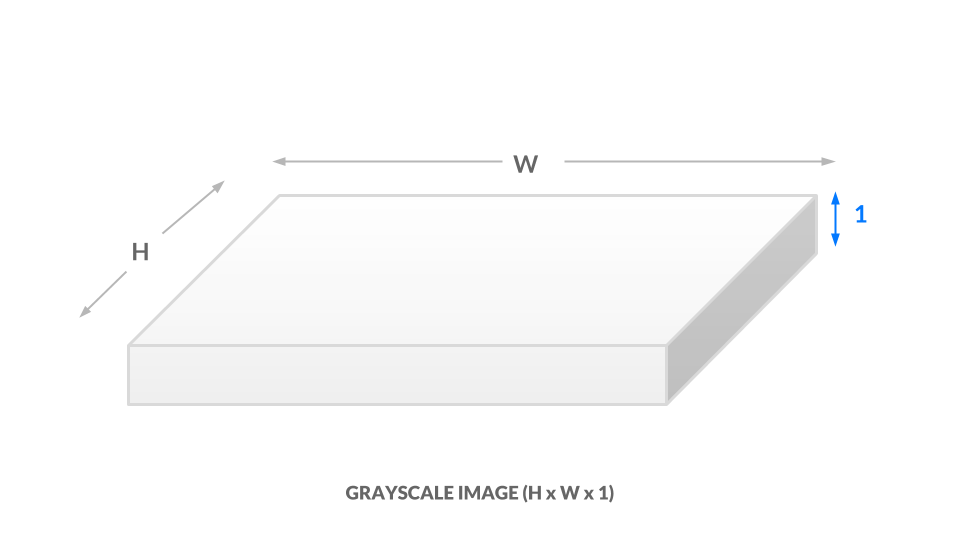For a color representation, we represent the color of each pixel using three values between 0 and 1. One number corresponds to the "degree of red," one to the "degree of green," and the last to the "degree of blue." We call this the RGB color space. This means that for every `(x, y)` position in our image, we have three values `(r, g, b)`. As a result, our image is now `h x w x 3`: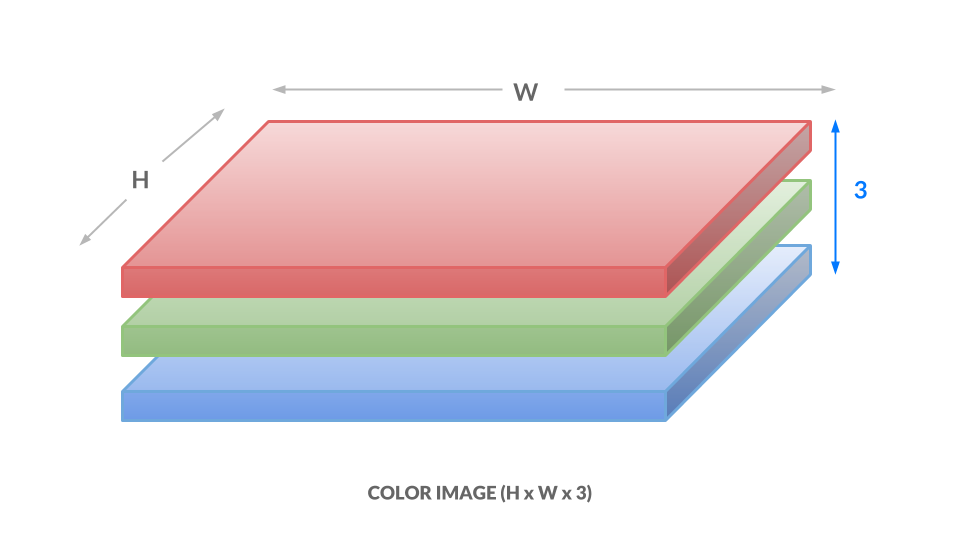Here, each number ranges from 0 to 255 instead of 0 to 1, but the idea is the same. Different combinations of numbers correspond to different colors, such as dark purple `(102, 0, 204)` or bright orange `(255, 153, 51)`. The takeaways are as follows:

1. Each image will be represented as a box of numbers that has three dimensions: height, width, and color channels. Manipulating this box of numbers directly is equivalent to manipulating the image.
2. We can also flatten this box to become just a list of numbers. In this way, our image becomes a vector. Later on, we will refer to images as vectors.

Now that you understand how images are represented numerically, you are well-equipped to begin applying dog masks to faces. To apply a dog mask, you will replace values in the child image with non-white dog mask pixels. To start, you will work with a single image. Download this crop of a face from the image you used in Step 2.

``````wget -O assets/child.png https://assets.digitalocean.com/articles/python3_dogfilter/alXjNK1.png
``````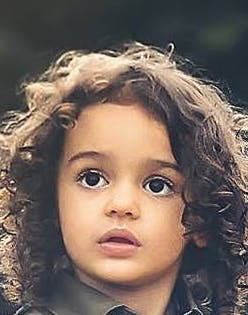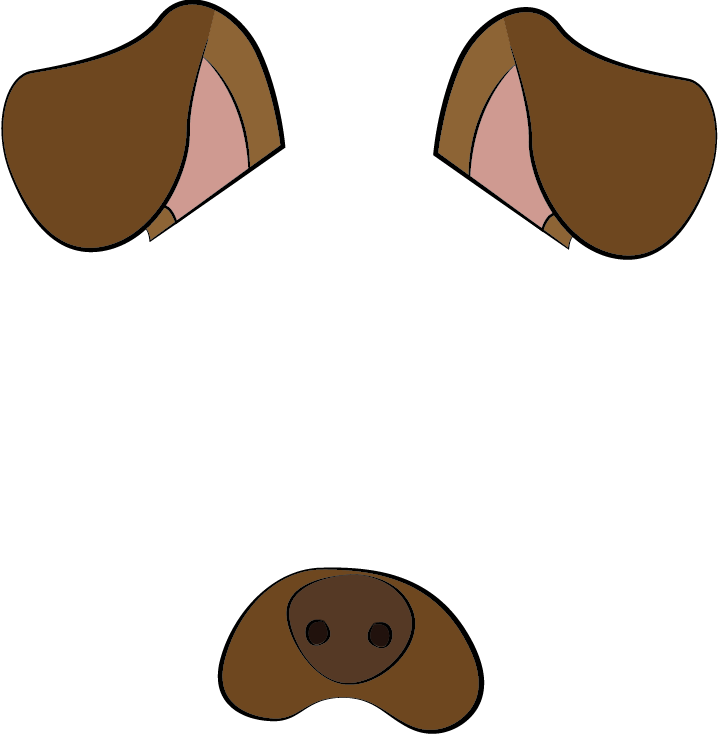Download this with `wget`:

``````wget -O assets/dog.png https://assets.digitalocean.com/articles/python3_dogfilter/ED32BCs.png
``````

Create a new file called `step_4_dog_mask_simple.py` which will hold the code for the script that applies the dog mask to faces:

``````nano step_4_dog_mask_simple.py
``````

Add the following boilerplate for the Python script and import the OpenCV and `numpy` libraries:

``````"""Test for adding dog mask"""

import cv2
import numpy as np

def main():
pass

if __name__ == '__main__':
main()
``````

Replace `pass` in the `main` function with these two lines which load the original image and the dog mask into memory.

``````...
def main():
``````

Next, fit the dog mask to the child. The logic is more complicated than what we've done previously, so we will create a new function called `apply_mask` to modularize our code. Directly after the two lines that load the images, add this line which invokes the `apply_mask` function:

``````...
``````

Create a new function called `apply_mask` and place it above the `main` function:

``````...
pass

def main():
...
``````

At this point, your file should look like this:

``````"""Test for adding dog mask"""

import cv2
import numpy as np

pass

def main():

if __name__ == '__main__':
main()
``````

Let's build out the `apply_mask` function. Our goal is to apply the mask to the child's face. However, we need to maintain the aspect ratio for our dog mask. To do so, we need to explicitly compute our dog mask's final dimensions. Inside the `apply_mask` function, replace `pass` with these two lines which extract the height and width of both images:

``````...
face_h, face_w, _ = face.shape
``````

Next, determine which dimension needs to be "shrunk more." To be precise, we need the tighter of the two constraints. Add this line to the `apply_mask` function:

``````...

# Resize the mask to fit on face
``````

Then compute the new shape by adding this code to the function:

``````...
``````

Here we cast the numbers to integers, as the `resize` function needs integral dimensions.

Now add this code to resize the dog mask to the new shape:

``````...

``````

Finally, write the image to disk so you can double-check that your resized dog mask is correct after you run the script:

``````    cv2.imwrite('outputs/resized_dog.png', resized_mask)
``````

The completed script should look like this:

``````"""Test for adding dog mask"""
import cv2
import numpy as np

face_h, face_w, _ = face.shape

# Resize the mask to fit on face

def main():

if __name__ == '__main__':
main()

``````

Save the file and exit your editor. Run the new script:

``````python step_4_dog_mask_simple.py
``````

Open the image at `outputs/resized_dog.png` to double-check the mask was resized correctly. It will match the dog mask shown earlier in this section.

Now add the dog mask to the child. Open the `step_4_dog_mask_simple.py` file again and return to the `apply_mask` function:

``````nano step_4_dog_mask_simple.py
``````

First, remove the line of code that writes the resized mask from the `apply_mask` function since you no longer need it:

``````    cv2.imwrite('outputs/resized_dog.png', resized_mask)  # delete this line
...
``````

In its place, apply your knowledge of image representation from the start of this section to modify the image. Start by making a copy of the child image. Add this line to the `apply_mask` function:

``````...
``````

Next, find all positions where the dog mask is not white or near white. To do this, check if the pixel value is less than 250 across all color channels, as we'd expect a near-white pixel to be near `[255, 255, 255]`. Add this code:

``````...
``````

At this point, the dog image is, at most, as large as the child image. We want to center the dog image on the face, so compute the offset needed to center the dog image by adding this code to `apply_mask`:

``````...
off_h = int((face_h - new_mask_h) / 2)
off_w = int((face_w - new_mask_w) / 2)
``````

Copy all non-white pixels from the dog image into the child image. Since the child image may be larger than the dog image, we need to take a subset of the child image:

``````    face_with_mask[off_h: off_h+new_mask_h, off_w: off_w+new_mask_w][non_white_pixels] = \
``````

Then return the result:

``````    return face_with_mask
``````

In the `main` function, add this code to write the result of the `apply_mask` function to an output image so you can manually double-check the result:

``````...
``````

Your completed script will look like the following:

``````"""Test for adding dog mask"""

import cv2
import numpy as np

face_h, face_w, _ = face.shape

# Resize the mask to fit on face

off_h = int((face_h - new_mask_h) / 2)
off_w = int((face_w - new_mask_w) / 2)

def main():

if __name__ == '__main__':
main()
``````

Save the script and run it:

``````python step_4_dog_mask_simple.py
``````

You'll have the following picture of a child with a dog mask in `outputs/child_with_dog_mask.png`: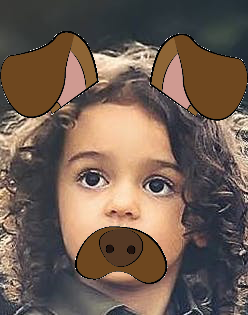You now have a utility that applies dog masks to faces. Now let's use what you've built to add the dog mask in real time.

We'll pick up from where we left off in Step 3. Copy `step_3_camera_face_detect.py` to `step_4_dog_mask.py`.

``````cp step_3_camera_face_detect.py step_4_dog_mask.py
``````

``````nano step_4_dog_mask.py
``````

First, import the NumPy library at the top of the script:

``````import numpy as np
...
``````

Then add the `apply_mask` function from your previous work into this new file above the `main` function:

``````def apply_mask(face: np.array, mask: np.array) -> np.array:
face_h, face_w, _ = face.shape

# Resize the mask to fit on face

off_h = int((face_h - new_mask_h) / 2)
off_w = int((face_w - new_mask_w) / 2)

...
``````

Second, locate this line in the `main` function:

``````    cap = cv2.VideoCapture(0)
``````

``````    cap = cv2.VideoCapture(0)

...
``````

Next, in the `while` loop, locate this line:

``````        ret, frame = cap.read()
``````

Add this line after it to extract the image's height and width:

``````        ret, frame = cap.read()
frame_h, frame_w, _ = frame.shape
...
``````

Next, delete the line in `main` that draws bounding boxes. You'll find this line in the `for` loop that iterates over detected faces:

``````        for x, y, w, h in rects:
...
cv2.rectangle(frame, (x, y), (x + w, y + h), (0, 255, 0), 2) # DELETE ME
...
``````

In its place, add this code which crops the frame. For aesthetic purposes, we crop an area slightly larger than the face.

``````        for x, y, w, h in rects:
# crop a frame slightly larger than the face
y0, y1 = int(y - 0.25*h), int(y + 0.75*h)
x0, x1 = x, x + w
``````

Introduce a check in case the detected face is too close to the edge.

``````            # give up if the cropped frame would be out-of-bounds
if x0 < 0 or y0 < 0 or x1 > frame_w or y1 > frame_h:
continue
``````

Finally, insert the face with a mask into the image.

``````            # apply mask
``````

Verify that your script looks like this:

``````"""Real-time dog filter

Move your face around and a dog filter will be applied to your face if it is not out-of-bounds. With the test frame in focus, hit `q` to exit. Note that typing `q` into your terminal will do nothing.
"""

import numpy as np
import cv2

face_h, face_w, _ = face.shape

# Resize the mask to fit on face

off_h = int((face_h - new_mask_h) / 2)
off_w = int((face_w - new_mask_w) / 2)

def main():
cap = cv2.VideoCapture(0)

# initialize front face classifier

while(True):
# Capture frame-by-frame
frame_h, frame_w, _ = frame.shape

# Convert to black-and-white
gray = cv2.cvtColor(frame, cv2.COLOR_BGR2GRAY)
blackwhite = cv2.equalizeHist(gray)

# Detect faces
blackwhite, scaleFactor=1.3, minNeighbors=4, minSize=(30, 30),

for x, y, w, h in rects:
# crop a frame slightly larger than the face
y0, y1 = int(y - 0.25*h), int(y + 0.75*h)
x0, x1 = x, x + w

# give up if the cropped frame would be out-of-bounds
if x0 < 0 or y0 < 0 or x1 > frame_w or y1 > frame_h:
continue

# Display the resulting frame
cv2.imshow('frame', frame)
if cv2.waitKey(1) & 0xFF == ord('q'):
break

# When everything done, release the capture
cap.release()
cv2.destroyAllWindows()

if __name__ == '__main__':
main()
``````

Save the file and exit your editor. Then run the script.

``````python step_4_dog_mask.py
``````

You now have a real-time dog filter running. The script will also work with multiple faces in the picture, so you can get your friends together for some automatic dog-ification.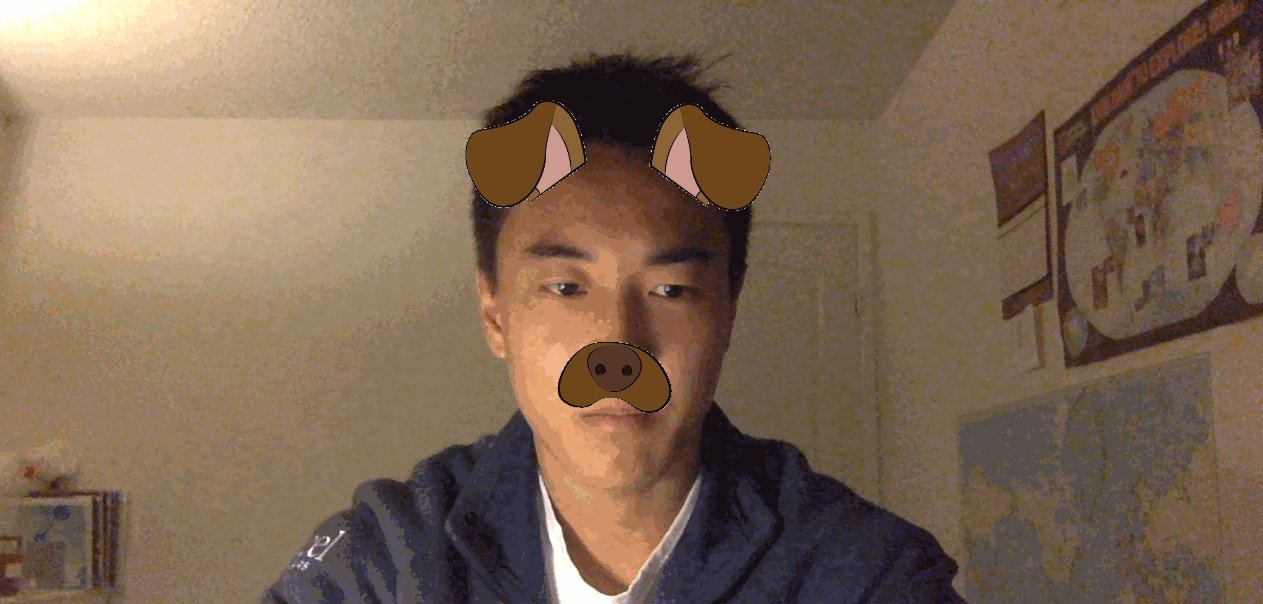This concludes our first primary objective in this tutorial, which is to create a Snapchat-esque dog filter. Now let's use facial expression to determine the dog mask applied to a face.

## Step 5 — Build a Basic Face Emotion Classifier using Least Squares

In this section you'll create an emotion classifier to apply different masks based on displayed emotions. If you smile, the filter will apply a corgi mask. If you frown, it will apply a pug mask. Along the way, you'll explore the least-squares framework, which is fundamental to understanding and discussing machine learning concepts.

To understand how to process our data and produce predictions, we'll first briefly explore machine learning models.

We need to ask two questions for each model that we consider. For now, these two questions will be sufficient to differentiate between models:

1. Input: What information is the model given?
2. Output: What is the model trying to predict?

At a high-level, the goal is to develop a model for emotion classification. The model is:

1. Input: given images of faces.
2. Output: predicts the corresponding emotion.
``````model: face -> emotion
``````

The approach we'll use is least squares; we take a set of points, and we find a line of best fit. The line of best fit, shown in the following image, is our model.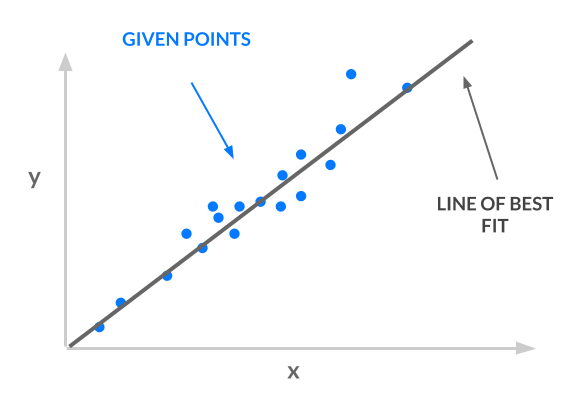Consider the input and output for our line:

1. Input: given `x` coordinates.
2. Output: predicts the corresponding \$y\$ coordinate.
``````least squares line: x -> y
``````

Our input `x` must represent faces and our output `y` must represent emotion, in order for us to use least squares for emotion classification:

• `x -> face`: Instead of using one number for `x`, we will use a vector of values for `x`. Thus, `x` can represent images of faces. The article Ordinary Least Squares explains why you can use a vector of values for `x`.
• `y -> emotion`: Each emotion will correspond to a number. For example, "angry" is 0, "sad" is 1, and "happy" is 2. In this way, `y` can represent emotions. However, our line is not constrained to output the `y` values 0, 1, and 2. It has an infinite number of possible y values–it could be 1.2, 3.5, or 10003.42. How do we translate those `y` values to integers corresponding to classes? See the article One-Hot Encoding for more detail and explanation.

Armed with this background knowledge, you will build a simple least-squares classifier using vectorized images and one-hot encoded labels. You'll accomplish this in three steps:

1. Preprocess the data: As explained at the start of this section, our samples are vectors where each vector encodes an image of a face. Our labels are integers corresponding to an emotion, and we'll apply one-hot encoding to these labels.
2. Specify and train the model: Use the closed-form least squares solution, `w^*`.
3. Run a prediction using the model: Take the argmax of `Xw^*` to obtain predicted emotions.

Let's get started.

First, set up a directory to contain the data:

``````mkdir data
``````

Then download the data, curated by Pierre-Luc Carrier and Aaron Courville, from a 2013 Face Emotion Classification competition on Kaggle.

``````wget -O data/fer2013.tar https://bitbucket.org/alvinwan/adversarial-examples-in-computer-vision-building-then-fooling/raw/babfe4651f89a398c4b3fdbdd6d7a697c5104cff/fer2013.tar
``````

Navigate to the `data` directory and unpack the data.

``````cd data
tar -xzf fer2013.tar
``````

Now we'll create a script to run the least-squares model. Navigate to the root of your project:

``````cd ~/DogFilter
``````

Create a new file for the script:

``````nano step_5_ls_simple.py
``````

Add Python boilerplate and import the packages you will need:

step_5_ls_simple.py

``````"""Train emotion classifier using least squares."""

import numpy as np

def main():
pass

if __name__ == '__main__':
main()
``````

Next, load the data into memory. Replace `pass` in your `main` function with the following code:

step_5_ls_simple.py

``````    # load data
X_train, Y_train = data['X'], data['Y']

X_test, Y_test = data['X'], data['Y']
``````

Now one-hot encode the labels. To do this, construct the identity matrix with `numpy` and then index into this matrix using our list of labels:

step_5_ls_simple.py

``````    # one-hot labels
I = np.eye(6)
Y_oh_train, Y_oh_test = I[Y_train], I[Y_test]
``````

Here, we use the fact that the `i`-th row in the identity matrix is all zero, except for the `i`-th entry. Thus, the i-th row is the one-hot encoding for the label of class `i`. Additionally, we use `numpy`'s advanced indexing, where `[a, b, c, d][[1, 3]] = [b, d]`.

Computing `(X^TX)^{-1}` would take too long on commodity hardware, as `X^TX` is a `2304x2304` matrix with over four million values, so we'll reduce this time by selecting only the first 100 features. Add this code:

step_5_ls_simple.py

``````...
# select first 100 dimensions
A_train, A_test = X_train[:, :100], X_test[:, :100]
``````

Next, add this code to evaluate the closed-form least-squares solution:

step_5_ls_simple.py

``````...
# train model
w = np.linalg.inv(A_train.T.dot(A_train)).dot(A_train.T.dot(Y_oh_train))
``````

Then define an evaluation function for training and validation sets. Place this before your `main` function:

step_5_ls_simple.py

``````def evaluate(A, Y, w):
Yhat = np.argmax(A.dot(w), axis=1)
return np.sum(Yhat == Y) / Y.shape
``````

To estimate labels, we take the inner product with each sample and get the indices of the maximum values using `np.argmax`. Then we compute the average number of correct classifications. This final number is your accuracy.

Finally, add this code to the end of the `main` function to compute the training and validation accuracy using the `evaluate` function you just wrote:

step_5_ls_simple.py

``````    # evaluate model
ols_train_accuracy = evaluate(A_train, Y_train, w)
print('(ols) Train Accuracy:', ols_train_accuracy)
ols_test_accuracy = evaluate(A_test, Y_test, w)
print('(ols) Test Accuracy:', ols_test_accuracy)
``````

Double-check that your script matches the following:

step_5_ls_simple.py

``````"""Train emotion classifier using least squares."""

import numpy as np

def evaluate(A, Y, w):
Yhat = np.argmax(A.dot(w), axis=1)
return np.sum(Yhat == Y) / Y.shape

def main():

X_train, Y_train = data['X'], data['Y']

X_test, Y_test = data['X'], data['Y']

# one-hot labels
I = np.eye(6)
Y_oh_train, Y_oh_test = I[Y_train], I[Y_test]

# select first 100 dimensions
A_train, A_test = X_train[:, :100], X_test[:, :100]

# train model
w = np.linalg.inv(A_train.T.dot(A_train)).dot(A_train.T.dot(Y_oh_train))

# evaluate model
ols_train_accuracy = evaluate(A_train, Y_train, w)
print('(ols) Train Accuracy:', ols_train_accuracy)
ols_test_accuracy = evaluate(A_test, Y_test, w)
print('(ols) Test Accuracy:', ols_test_accuracy)

if __name__ == '__main__':
main()
``````

``````python step_5_ls_simple.py
``````

You'll see the following output:

``````Output
(ols) Train Accuracy: 0.4748918316507146
(ols) Test Accuracy: 0.45280545359202934
``````

Our model gives 47.5% train accuracy. We repeat this on the validation set to obtain 45.3% accuracy. For a three-way classification problem, 45.3% is reasonably above guessing, which is 33%​. This is our starting classifier for emotion detection, and in the next step, you'll build off of this least-squares model to improve accuracy. The higher the accuracy, the more reliably your emotion-based dog filter can find the appropriate dog filter for each detected emotion.

## Step 6 — Improving Accuracy by Featurizing the Inputs

We can use a more expressive model to boost accuracy. To accomplish this, we featurize our inputs.

The original image tells us that position (`0, 0`) is red, (`1, 0`) is brown, and so on. A featurized image may tell us that there is a dog to the top-left of the image, a person in the middle, etc. Featurization is powerful, but its precise definition is beyond the scope of this tutorial.

We'll use an approximation for the radial basis function (RBF) kernel, using a random Gaussian matrix. We won't go into detail in this tutorial. Instead, we'll treat this as a black box that computes higher-order features for us.

We'll continue where we left off in the previous step. Copy the previous script so you have a good starting point:

``````cp step_5_ls_simple.py step_6_ls_simple.py
``````

Open the new file in your editor:

``````nano step_6_ls_simple.py
``````

We'll start by creating the featurizing random matrix. Again, we'll use only 100 features in our new feature space.

Locate the following line, defining `A_train` and `A_test`:

step_6_ls_simple.py

``````    # select first 100 dimensions
A_train, A_test = X_train[:, :100], X_test[:, :100]
``````

Directly above this definition for `A_train` and `A_test`, add a random feature matrix:

step_6_ls_simple.py

``````    d = 100
W = np.random.normal(size=(X_train.shape, d))
# select first 100 dimensions
A_train, A_test = X_train[:, :100], X_test[:, :100]  ...
``````

Then replace the definitions for `A_train` and `A_test`. We redefine our matrices, called design matrices, using this random featurization.

step_6_ls_simple.py

``````    A_train, A_test = X_train.dot(W), X_test.dot(W)
``````

Save your file and run the script.

``````python step_6_ls_simple.py
``````

You'll see the following output:

``````Output
(ols) Train Accuracy: 0.584174642717
(ols) Test Accuracy: 0.584425799685
``````

This featurization now offers 58.4% train accuracy and 58.4% validation accuracy, a 13.1% improvement in validation results. We trimmed the X matrix to be `100 x 100`, but the choice of 100 was arbirtary. We could also trim the `X` matrix to be `1000 x 1000` or `50 x 50`. Say the dimension of `x` is `d x d`. We can test more values of `d` by re-trimming X to be `d x d` and recomputing a new model.

Trying more values of `d`, we find an additional 4.3% improvement in test accuracy to 61.7%. In the following figure, we consider the performance of our new classifier as we vary `d`. Intuitively, as `d` increases, the accuracy should also increase, as we use more and more of our original data. Rather than paint a rosy picture, however, the graph exhibits a negative trend: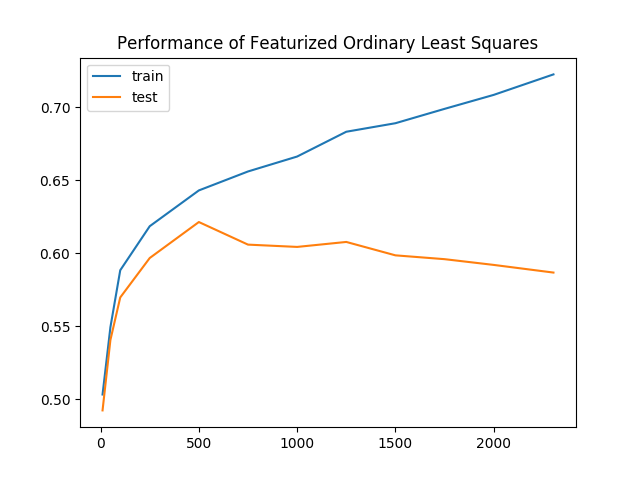As we keep more of our data, the gap between the training and validation accuracies increases as well. This is clear evidence of overfitting, where our model is learning representations that are no longer generalizable to all data. To combat overfitting, we'll regularize our model by penalizing complex models.

We amend our ordinary least-squares objective function with a regularization term, giving us a new objective. Our new objective function is called ridge regression and it looks like this:

``````min_w |Aw- y|^2 + lambda |w|^2
``````

In this equation, `lambda` is a tunable hyperparameter. Plug `lambda = 0` into the equation and ridge regression becomes least-squares. Plug `lambda = infinity` into the equation, and you'll find the best `w` must now be zero, as any non-zero `w` incurs infinite loss. As it turns out, this objective yields a closed-form solution as well:

``````w^* = (A^TA + lambda I)^{-1}A^Ty
``````

Still using the featurized samples, retrain and reevaluate the model once more.

Open `step_6_ls_simple.py` again in your editor:

``````nano step_6_ls_simple.py
``````

This time, increase the dimensionality of the new feature space to `d=1000​`. Change the value of `d` from `100` to `1000` as shown in the following code block:

step_6_ls_simple.py

``````...
d = 1000
W = np.random.normal(size=(X_train.shape, d))
...
``````

Then apply ridge regression using a regularization of `lambda = 10^{10}`. Replace the line defining `w` with the following two lines:

step_6_ls_simple.py

``````...
# train model
I = np.eye(A_train.shape)
w = np.linalg.inv(A_train.T.dot(A_train) + 1e10 * I).dot(A_train.T.dot(Y_oh_train))
``````

Then locate this block:

step_6_ls_simple.py

``````...
ols_train_accuracy = evaluate(A_train, Y_train, w)
print('(ols) Train Accuracy:', ols_train_accuracy)
ols_test_accuracy = evaluate(A_test, Y_test, w)
print('(ols) Test Accuracy:', ols_test_accuracy)
``````

Replace it with the following:

step_6_ls_simple.py

``````...

print('(ridge) Train Accuracy:', evaluate(A_train, Y_train, w))
print('(ridge) Test Accuracy:', evaluate(A_test, Y_test, w))
``````

The completed script should look like this:

step_6_ls_simple.py

``````"""Train emotion classifier using least squares."""

import numpy as np

def evaluate(A, Y, w):
Yhat = np.argmax(A.dot(w), axis=1)
return np.sum(Yhat == Y) / Y.shape

def main():
X_train, Y_train = data['X'], data['Y']

X_test, Y_test = data['X'], data['Y']

# one-hot labels
I = np.eye(6)
Y_oh_train, Y_oh_test = I[Y_train], I[Y_test]
d = 1000
W = np.random.normal(size=(X_train.shape, d))
# select first 100 dimensions
A_train, A_test = X_train.dot(W), X_test.dot(W)

# train model
I = np.eye(A_train.shape)
w = np.linalg.inv(A_train.T.dot(A_train) + 1e10 * I).dot(A_train.T.dot(Y_oh_train))

# evaluate model
print('(ridge) Train Accuracy:', evaluate(A_train, Y_train, w))
print('(ridge) Test Accuracy:', evaluate(A_test, Y_test, w))

if __name__ == '__main__':
main()
``````

Save the file, exit your editor, and run the script:

``````python step_6_ls_simple.py
``````

You'll see the following output:

``````Output
(ridge) Train Accuracy: 0.651173462698
(ridge) Test Accuracy: 0.622181436812
``````

There's an additional improvement of 0.4% in validation accuracy to 62.2%, as train accuracy drops to 65.1%. Once again reevaluating across a number of different `d`, we see a smaller gap between training and validation accuracies for ridge regression. In other words, ridge regression was subject to less overfitting.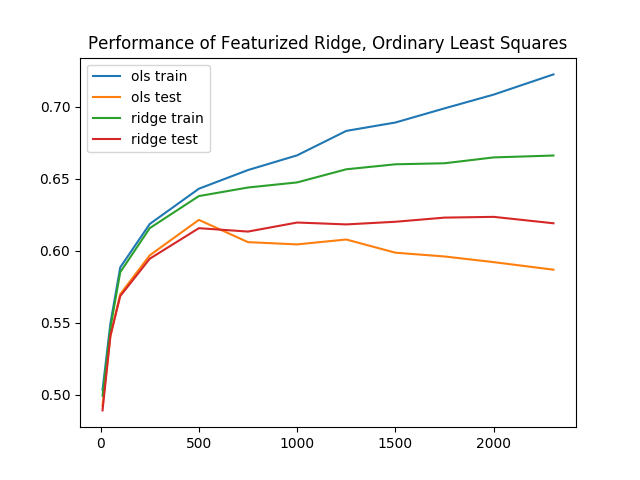Baseline performance for least squares, with these extra enhancements, performs reasonably well. The training and inference times, all together, take no more than 20 seconds for even the best results. In the next section, you'll explore even more complex models.

## Step 7 — Building the Face-Emotion Classifier Using a Convolutional Neural Network in PyTorch

In this section, you'll build a second emotion classifier using neural networks instead of least squares. Again, our goal is to produce a model that accepts faces as input and outputs an emotion. Eventually, this classifier will then determine which dog mask to apply.

For a brief neural network visualization and introduction, see the article Understanding Neural Networks. Here, we will use a deep-learning library called PyTorch. There are a number of deep-learning libraries in widespread use, and each has various pros and cons. PyTorch is a particularly good place to start. To impliment this neural network classifier, we again take three steps, as we did with the least-squares classifier:

1. Preprocess the data: Apply one-hot encoding and then apply PyTorch abstractions.
2. Specify and train the model: Set up a neural network using PyTorch layers. Define optimization hyperparameters and run stochastic gradient descent.
3. Run a prediction using the model: Evaluate the neural network.

Create a new file, named `step_7_fer_simple.py`

``````nano step_7_fer_simple.py
``````

Import the necessary utilities and create a Python class that will hold your data. For data processing here, you will create the train and test datasets. To do these, implement PyTorch's `Dataset` interface, which lets you load and use PyTorch's built-in data pipeline for the face-emotion recognition dataset:

step_7_fer_simple.py

``````from torch.utils.data import Dataset
import torch.nn as nn
import torch.nn.functional as F
import torch.optim as optim
import numpy as np
import torch
import cv2
import argparse

class Fer2013Dataset(Dataset):
"""Face Emotion Recognition dataset.

and Aaron Courville in 2013.

Each sample is 1 x 1 x 48 x 48, and each label is a scalar.
"""
pass
``````

Delete the `pass` placeholder in the `Fer2013Dataset` class. In its place, add a function that will initialize our data holder:

step_7_fer_simple.py

``````    def __init__(self, path: str):
"""
Args:
path: Path to `.np` file containing sample nxd and label nx1
"""
self._samples = data['X']
self._labels = data['Y']
self._samples = self._samples.reshape((-1, 1, 48, 48))

self.X = Variable(torch.from_numpy(self._samples)).float()
self.Y = Variable(torch.from_numpy(self._labels)).float()
...
``````

This function starts by loading the samples and labels. Then it wraps the data in PyTorch data structures.

Directly after the `__init__` function, add a `__len__` function, as this is needed to implement the `Dataset` interface PyTorch expects:

step_7_fer_simple.py

``````...
def __len__(self):
return len(self._labels)
``````

Finally, add a `__getitem__` method, which returns a dictionary containing the sample and the label:

step_7_fer_simple.py

``````    def __getitem__(self, idx):
return {'image': self._samples[idx], 'label': self._labels[idx]}
``````

Double-check that your file looks like the following:

step_7_fer_simple.py

``````from torch.utils.data import Dataset
import torch.nn as nn
import torch.nn.functional as F
import torch.optim as optim
import numpy as np
import torch
import cv2
import argparse

class Fer2013Dataset(Dataset):
"""Face Emotion Recognition dataset.
and Aaron Courville in 2013.
Each sample is 1 x 1 x 48 x 48, and each label is a scalar.
"""

def __init__(self, path: str):
"""
Args:
path: Path to `.np` file containing sample nxd and label nx1
"""
self._samples = data['X']
self._labels = data['Y']
self._samples = self._samples.reshape((-1, 1, 48, 48))

self.X = Variable(torch.from_numpy(self._samples)).float()
self.Y = Variable(torch.from_numpy(self._labels)).float()

def __len__(self):
return len(self._labels)

def __getitem__(self, idx):
return {'image': self._samples[idx], 'label': self._labels[idx]}
``````

Next, load the `Fer2013Dataset` dataset. Add the following code to the end of your file after the `Fer2013Dataset` class:

step_7_fer_simple.py

``````trainset = Fer2013Dataset('data/fer2013_train.npz')

testset = Fer2013Dataset('data/fer2013_test.npz')
``````

This code initializes the dataset using the `Fer2013Dataset` class you created. Then for the train and validation sets, it wraps the dataset in a `DataLoader`. This translates the dataset into an iterable to use later.

As a sanity check, verify that the dataset utilities are functioning. Create a sample dataset loader using `DataLoader` and print the first element of that loader. Add the following to the end of your file:

step_7_fer_simple.py

``````if __name__ == '__main__':
``````

Verify that your completed script looks like this:

step_7_fer_simple.py

``````from torch.utils.data import Dataset
import torch.nn as nn
import torch.nn.functional as F
import torch.optim as optim
import numpy as np
import torch
import cv2
import argparse

class Fer2013Dataset(Dataset):
"""Face Emotion Recognition dataset.
and Aaron Courville in 2013.
Each sample is 1 x 1 x 48 x 48, and each label is a scalar.
"""

def __init__(self, path: str):
"""
Args:
path: Path to `.np` file containing sample nxd and label nx1
"""
self._samples = data['X']
self._labels = data['Y']
self._samples = self._samples.reshape((-1, 1, 48, 48))

self.X = Variable(torch.from_numpy(self._samples)).float()
self.Y = Variable(torch.from_numpy(self._labels)).float()

def __len__(self):
return len(self._labels)

def __getitem__(self, idx):
return {'image': self._samples[idx], 'label': self._labels[idx]}

trainset = Fer2013Dataset('data/fer2013_train.npz')

testset = Fer2013Dataset('data/fer2013_test.npz')

if __name__ == '__main__':
``````

Exit your editor and run the script.

``````python step_7_fer_simple.py
``````

This outputs the following pair of tensors. Our data pipeline outputs two samples and two labels. This indicates that our data pipeline is up and ready to go:

``````Output
{'image':
(0 ,0 ,.,.) =
24   32   36  ...   173  172  173
25   34   29  ...   173  172  173
26   29   25  ...   172  172  174
...         ⋱        ...
159  185  157  ...   157  156  153
136  157  187  ...   152  152  150
145  130  161  ...   142  143  142
⋮

(1 ,0 ,.,.) =
20   17   19  ...   187  176  162
22   17   17  ...   195  180  171
17   17   18  ...   203  193  175
...         ⋱        ...
1    1    1  ...   106  115  119
2    2    1  ...   103  111  119
2    2    2  ...    99  107  118
[torch.LongTensor of size 2x1x48x48]
, 'label':
1
1
[torch.LongTensor of size 2]
}
``````

Now that you've verified that the data pipeline works, return to `step_7_fer_simple.py` to add the neural network and optimizer. Open `step_7_fer_simple.py`.

``````nano step_7_fer_simple.py
``````

First, delete the last three lines you added in the previous iteration:

step_7_fer_simple.py

``````# Delete all three lines
if __name__ == '__main__':
``````

In their place, define a PyTorch neural network that includes three convolutional layers, followed by three fully connected layers. Add this to the end of your existing script:

step_7_fer_simple.py

``````class Net(nn.Module):
def __init__(self):
super(Net, self).__init__()
self.conv1 = nn.Conv2d(1, 6, 5)
self.pool = nn.MaxPool2d(2, 2)
self.conv2 = nn.Conv2d(6, 6, 3)
self.conv3 = nn.Conv2d(6, 16, 3)
self.fc1 = nn.Linear(16 * 4 * 4, 120)
self.fc2 = nn.Linear(120, 48)
self.fc3 = nn.Linear(48, 3)

def forward(self, x):
x = self.pool(F.relu(self.conv1(x)))
x = self.pool(F.relu(self.conv2(x)))
x = self.pool(F.relu(self.conv3(x)))
x = x.view(-1, 16 * 4 * 4)
x = F.relu(self.fc1(x))
x = F.relu(self.fc2(x))
x = self.fc3(x)
return x
``````

Now initialize the neural network, define a loss function, and define optimization hyperparameters by adding the following code to the end of the script:

step_7_fer_simple.py

``````net = Net().float()
criterion = nn.CrossEntropyLoss()
optimizer = optim.SGD(net.parameters(), lr=0.001, momentum=0.9)
``````

We'll train for two epochs. For now, we define an epoch to be an iteration of training where every training sample has been used exactly once.

First, extract `image` and `label` from the dataset loader and then wrap each in a PyTorch `Variable`. Second, run the forward pass and then backpropagate through the loss and neural network. Add the following code to the end of your script to do that:

step_7_fer_simple.py

``````for epoch in range(2):  # loop over the dataset multiple times

running_loss = 0.0
for i, data in enumerate(trainloader, 0):
inputs = Variable(data['image'].float())
labels = Variable(data['label'].long())

# forward + backward + optimize
outputs = net(inputs)
loss = criterion(outputs, labels)
loss.backward()
optimizer.step()

# print statistics
running_loss += loss.data
if i % 100 == 0:
print('[%d, %5d] loss: %.3f' % (epoch, i, running_loss / (i + 1)))
``````

Your script should now look like this:

step_7_fer_simple.py

``````from torch.utils.data import Dataset
import torch.nn as nn
import torch.nn.functional as F
import torch.optim as optim
import numpy as np
import torch
import cv2
import argparse

class Fer2013Dataset(Dataset):
"""Face Emotion Recognition dataset.

and Aaron Courville in 2013.

Each sample is 1 x 1 x 48 x 48, and each label is a scalar.
"""
def __init__(self, path: str):
"""
Args:
path: Path to `.np` file containing sample nxd and label nx1
"""
self._samples = data['X']
self._labels = data['Y']
self._samples = self._samples.reshape((-1, 1, 48, 48))

self.X = Variable(torch.from_numpy(self._samples)).float()
self.Y = Variable(torch.from_numpy(self._labels)).float()

def __len__(self):
return len(self._labels)

def __getitem__(self, idx):
return {'image': self._samples[idx], 'label': self._labels[idx]}

trainset = Fer2013Dataset('data/fer2013_train.npz')

testset = Fer2013Dataset('data/fer2013_test.npz')

class Net(nn.Module):
def __init__(self):
super(Net, self).__init__()
self.conv1 = nn.Conv2d(1, 6, 5)
self.pool = nn.MaxPool2d(2, 2)
self.conv2 = nn.Conv2d(6, 6, 3)
self.conv3 = nn.Conv2d(6, 16, 3)
self.fc1 = nn.Linear(16 * 4 * 4, 120)
self.fc2 = nn.Linear(120, 48)
self.fc3 = nn.Linear(48, 3)

def forward(self, x):
x = self.pool(F.relu(self.conv1(x)))
x = self.pool(F.relu(self.conv2(x)))
x = self.pool(F.relu(self.conv3(x)))
x = x.view(-1, 16 * 4 * 4)
x = F.relu(self.fc1(x))
x = F.relu(self.fc2(x))
x = self.fc3(x)
return x

net = Net().float()
criterion = nn.CrossEntropyLoss()
optimizer = optim.SGD(net.parameters(), lr=0.001, momentum=0.9)

for epoch in range(2):  # loop over the dataset multiple times

running_loss = 0.0
for i, data in enumerate(trainloader, 0):
inputs = Variable(data['image'].float())
labels = Variable(data['label'].long())

# forward + backward + optimize
outputs = net(inputs)
loss = criterion(outputs, labels)
loss.backward()
optimizer.step()

# print statistics
running_loss += loss.data
if i % 100 == 0:
print('[%d, %5d] loss: %.3f' % (epoch, i, running_loss / (i + 1)))
``````

Save the file and exit the editor once you've verified your code. Then, launch this proof-of-concept training:

``````python step_7_fer_simple.py
``````

You'll see output similar to the following as the neural network trains:

``````Output
[0,     0] loss: 1.094
[0,   100] loss: 1.049
[0,   200] loss: 1.009
[0,   300] loss: 0.963
[0,   400] loss: 0.935
[1,     0] loss: 0.760
[1,   100] loss: 0.768
[1,   200] loss: 0.775
[1,   300] loss: 0.776
[1,   400] loss: 0.767
``````

You can then augment this script using a number of other PyTorch utilities to save and load models, output training and validation accuracies, fine-tune a learning-rate schedule, etc. After training for 20 epochs with a learning rate of 0.01 and momentum of 0.9, our neural network attains a 87.9% train accuracy and a 75.5% validation accuracy, a further 6.8% improvement over the most successful least-squares approach thus far at 66.6%. We'll include these additional bells and whistles in a new script.

Create a new file to hold the final face emotion detector which your live camera feed will use. This script contains the code above along with a command-line interface and an easy-to-import version of our code that will be used later. Additionally, it contains the hyperparameters tuned in advance, for a model with higher accuracy.

``````nano step_7_fer.py
``````

Start with the following imports. This matches our previous file but additionally includes OpenCV as `import cv2.`

step_7_fer.py

``````from torch.utils.data import Dataset
import torch.nn as nn
import torch.nn.functional as F
import torch.optim as optim
import numpy as np
import torch
import cv2
import argparse
``````

Directly beneath these imports, reuse your code from `step_7_fer_simple.py` to define the neural network:

step_7_fer.py

``````class Net(nn.Module):
def __init__(self):
super(Net, self).__init__()
self.conv1 = nn.Conv2d(1, 6, 5)
self.pool = nn.MaxPool2d(2, 2)
self.conv2 = nn.Conv2d(6, 6, 3)
self.conv3 = nn.Conv2d(6, 16, 3)
self.fc1 = nn.Linear(16 * 4 * 4, 120)
self.fc2 = nn.Linear(120, 48)
self.fc3 = nn.Linear(48, 3)

def forward(self, x):
x = self.pool(F.relu(self.conv1(x)))
x = self.pool(F.relu(self.conv2(x)))
x = self.pool(F.relu(self.conv3(x)))
x = x.view(-1, 16 * 4 * 4)
x = F.relu(self.fc1(x))
x = F.relu(self.fc2(x))
x = self.fc3(x)
return x
``````

Again, reuse the code for the Face Emotion Recognition dataset from `step_7_fer_simple.py` and add it to this file:

step_7_fer.py

``````class Fer2013Dataset(Dataset):
"""Face Emotion Recognition dataset.
and Aaron Courville in 2013.
Each sample is 1 x 1 x 48 x 48, and each label is a scalar.
"""

def __init__(self, path: str):
"""
Args:
path: Path to `.np` file containing sample nxd and label nx1
"""
self._samples = data['X']
self._labels = data['Y']
self._samples = self._samples.reshape((-1, 1, 48, 48))

self.X = Variable(torch.from_numpy(self._samples)).float()
self.Y = Variable(torch.from_numpy(self._labels)).float()

def __len__(self):
return len(self._labels)

def __getitem__(self, idx):
return {'image': self._samples[idx], 'label': self._labels[idx]}
``````

Next, define a few utilities to evaluate the neural network's performance. First, add an `evaluate` function which compares the neural network's predicted emotion to the true emotion for a single image:

step_7_fer.py

``````def evaluate(outputs: Variable, labels: Variable, normalized: bool=True) -> float:
"""Evaluate neural network outputs against non-one-hotted labels."""
Y = labels.data.numpy()
Yhat = np.argmax(outputs.data.numpy(), axis=1)
denom = Y.shape if normalized else 1
return float(np.sum(Yhat == Y) / denom)
``````

Then add a function called `batch_evaluate` which applies the first function to all images:

step_7_fer.py

``````def batch_evaluate(net: Net, dataset: Dataset, batch_size: int=500) -> float:
"""Evaluate neural network in batches, if dataset is too large."""
score = 0.0
n = dataset.X.shape
for i in range(0, n, batch_size):
x = dataset.X[i: i + batch_size]
y = dataset.Y[i: i + batch_size]
score += evaluate(net(x), y, False)
return score / n
``````

Now, define a function called `get_image_to_emotion_predictor` that takes in an image and outputs a predicted emotion, using a pretrained model:

step_7_fer.py

``````def get_image_to_emotion_predictor(model_path='assets/model_best.pth'):
"""Returns predictor, from image to emotion index."""
net = Net().float()

def predictor(image: np.array):
"""Translates images into emotion indices."""
if image.shape > 1:
image = cv2.cvtColor(image, cv2.COLOR_BGR2GRAY)
frame = cv2.resize(image, (48, 48)).reshape((1, 1, 48, 48))
X = Variable(torch.from_numpy(frame)).float()
return np.argmax(net(X).data.numpy(), axis=1)
return predictor
``````

Finally, add the following code to define the `main` function to leverage the other utilities:

step_7_fer.py

``````def main():
trainset = Fer2013Dataset('data/fer2013_train.npz')
testset = Fer2013Dataset('data/fer2013_test.npz')
net = Net().float()

train_acc = batch_evaluate(net, trainset, batch_size=500)
print('Training accuracy: %.3f' % train_acc)
test_acc = batch_evaluate(net, testset, batch_size=500)
print('Validation accuracy: %.3f' % test_acc)

if __name__ == '__main__':
main()
``````

This loads a pretrained neural network and evaluates its performance on the provided Face Emotion Recognition dataset. Specifically, the script outputs accuracy on the images we used for training, as well as a separate set of images we put aside for testing purposes.

Double-check that your file matches the following:

step_7_fer.py

``````from torch.utils.data import Dataset
import torch.nn as nn
import torch.nn.functional as F
import torch.optim as optim
import numpy as np
import torch
import cv2
import argparse

class Net(nn.Module):
def __init__(self):
super(Net, self).__init__()
self.conv1 = nn.Conv2d(1, 6, 5)
self.pool = nn.MaxPool2d(2, 2)
self.conv2 = nn.Conv2d(6, 6, 3)
self.conv3 = nn.Conv2d(6, 16, 3)
self.fc1 = nn.Linear(16 * 4 * 4, 120)
self.fc2 = nn.Linear(120, 48)
self.fc3 = nn.Linear(48, 3)

def forward(self, x):
x = self.pool(F.relu(self.conv1(x)))
x = self.pool(F.relu(self.conv2(x)))
x = self.pool(F.relu(self.conv3(x)))
x = x.view(-1, 16 * 4 * 4)
x = F.relu(self.fc1(x))
x = F.relu(self.fc2(x))
x = self.fc3(x)
return x

class Fer2013Dataset(Dataset):
"""Face Emotion Recognition dataset.
and Aaron Courville in 2013.
Each sample is 1 x 1 x 48 x 48, and each label is a scalar.
"""

def __init__(self, path: str):
"""
Args:
path: Path to `.np` file containing sample nxd and label nx1
"""
self._samples = data['X']
self._labels = data['Y']
self._samples = self._samples.reshape((-1, 1, 48, 48))

self.X = Variable(torch.from_numpy(self._samples)).float()
self.Y = Variable(torch.from_numpy(self._labels)).float()

def __len__(self):
return len(self._labels)

def __getitem__(self, idx):
return {'image': self._samples[idx], 'label': self._labels[idx]}

def evaluate(outputs: Variable, labels: Variable, normalized: bool=True) -> float:
"""Evaluate neural network outputs against non-one-hotted labels."""
Y = labels.data.numpy()
Yhat = np.argmax(outputs.data.numpy(), axis=1)
denom = Y.shape if normalized else 1
return float(np.sum(Yhat == Y) / denom)

def batch_evaluate(net: Net, dataset: Dataset, batch_size: int=500) -> float:
"""Evaluate neural network in batches, if dataset is too large."""
score = 0.0
n = dataset.X.shape
for i in range(0, n, batch_size):
x = dataset.X[i: i + batch_size]
y = dataset.Y[i: i + batch_size]
score += evaluate(net(x), y, False)
return score / n

def get_image_to_emotion_predictor(model_path='assets/model_best.pth'):
"""Returns predictor, from image to emotion index."""
net = Net().float()

def predictor(image: np.array):
"""Translates images into emotion indices."""
if image.shape > 1:
image = cv2.cvtColor(image, cv2.COLOR_BGR2GRAY)
frame = cv2.resize(image, (48, 48)).reshape((1, 1, 48, 48))
X = Variable(torch.from_numpy(frame)).float()
return np.argmax(net(X).data.numpy(), axis=1)
return predictor

def main():
trainset = Fer2013Dataset('data/fer2013_train.npz')
testset = Fer2013Dataset('data/fer2013_test.npz')
net = Net().float()

train_acc = batch_evaluate(net, trainset, batch_size=500)
print('Training accuracy: %.3f' % train_acc)
test_acc = batch_evaluate(net, testset, batch_size=500)
print('Validation accuracy: %.3f' % test_acc)

if __name__ == '__main__':
main(
``````

Save the file and exit your editor.

As before, with the face detector, download pre-trained model parameters and save them to your `assets` folder with the following command:

``````wget -O assets/model_best.pth https://github.com/alvinwan/emotion-based-dog-filter/raw/master/src/assets/model_best.pth
``````

Run the script to use and evaluate the pre-trained model:

``````python step_7_fer.py
``````

This will output the following:

``````Output
Training accuracy: 0.879
Validation accuracy: 0.755
``````

At this point, you've built a pretty accurate face-emotion classifier. In essence, our model can correctly disambiguate between faces that are happy, sad, and surprised eight out of ten times. This is a reasonably good model, so you can now move on to using this face-emotion classifier to determine which dog mask to apply to faces.

## Step 8 — Finishing the Emotion-Based Dog Filter

Before integrating our brand-new face-emotion classifier, we will need animal masks to pick from. We'll use a Dalmation mask and a Sheepdog mask: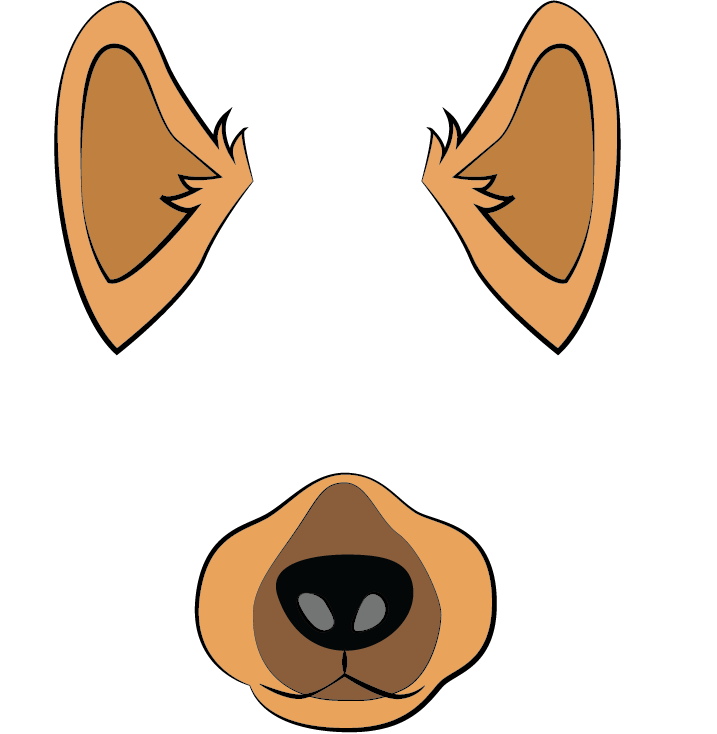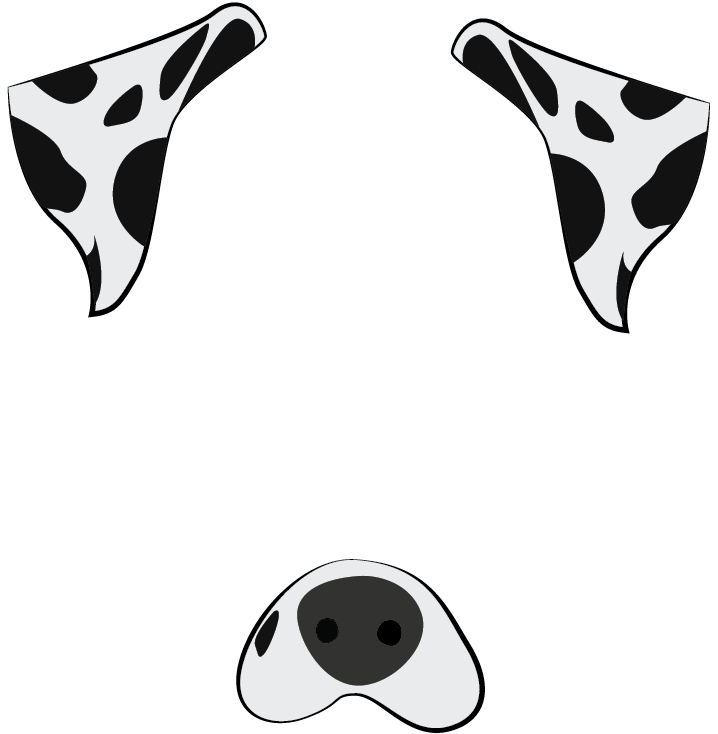Execute these commands to download both masks to your `assets` folder:

``````wget -O assets/dalmation.png https://assets.digitalocean.com/articles/python3_dogfilter/E9ax7PI.png  # dalmation
wget -O assets/sheepdog.png https://assets.digitalocean.com/articles/python3_dogfilter/HveFdkg.png  # sheepdog
``````

Now let's use the masks in our filter. Start by duplicating the `step_4_dog_mask.py` file:

``````cp step_4_dog_mask.py step_8_dog_emotion_mask.py
``````

Open the new Python script.

``````nano step_8_dog_emotion_mask.py
``````

Insert a new line at the top of the script to import the emotion predictor:

``````from step_7_fer import get_image_to_emotion_predictor
...
``````

Then, in the `main()` function, locate this line:

``````    mask = cv2.imread('assets/dog.png')
``````

Replace it with the following to load the new masks and aggregate all masks into a tuple:

``````    mask0 = cv2.imread('assets/dog.png')
``````

Add a line break, and then add this code to create the emotion predictor.

``````    # get emotion predictor
predictor = get_image_to_emotion_predictor()
``````

Your `main` function should now match the following:

``````def main():
cap = cv2.VideoCapture(0)

# get emotion predictor
predictor = get_image_to_emotion_predictor()

# initialize front face classifier
...
``````

Next, locate these lines:

``````            # apply mask
``````

Insert the following line below the `# apply mask` line to select the appropriate mask by using the predictor:

``````            # apply mask

``````

The completed file should look like this:

``````"""Test for face detection"""

from step_7_fer import get_image_to_emotion_predictor
import numpy as np
import cv2

face_h, face_w, _ = face.shape

# Resize the mask to fit on face

off_h = int((face_h - new_mask_h) / 2)
off_w = int((face_w - new_mask_w) / 2)

def main():

cap = cv2.VideoCapture(0)

# get emotion predictor
predictor = get_image_to_emotion_predictor()

# initialize front face classifier

while True:
# Capture frame-by-frame
frame_h, frame_w, _ = frame.shape

# Convert to black-and-white
gray = cv2.cvtColor(frame, cv2.COLOR_BGR2GRAY)
blackwhite = cv2.equalizeHist(gray)

blackwhite, scaleFactor=1.3, minNeighbors=4, minSize=(30, 30),

for x, y, w, h in rects:
# crop a frame slightly larger than the face
y0, y1 = int(y - 0.25*h), int(y + 0.75*h)
x0, x1 = x, x + w
# give up if the cropped frame would be out-of-bounds
if x0 < 0 or y0 < 0 or x1 > frame_w or y1 > frame_h:
continue

# Display the resulting frame
cv2.imshow('frame', frame)
if cv2.waitKey(1) & 0xFF == ord('q'):
break

cap.release()
cv2.destroyAllWindows()

if __name__ == '__main__':
main()
``````

Save and exit your editor. Now launch the script:

``````python step_8_dog_emotion_mask.py
``````

Now try it out! Smiling will register as "happy" and show the original dog. A neutral face or a frown will register as "sad" and yield the dalmation. A face of "surprise," with a nice big jaw drop, will yield the sheepdog.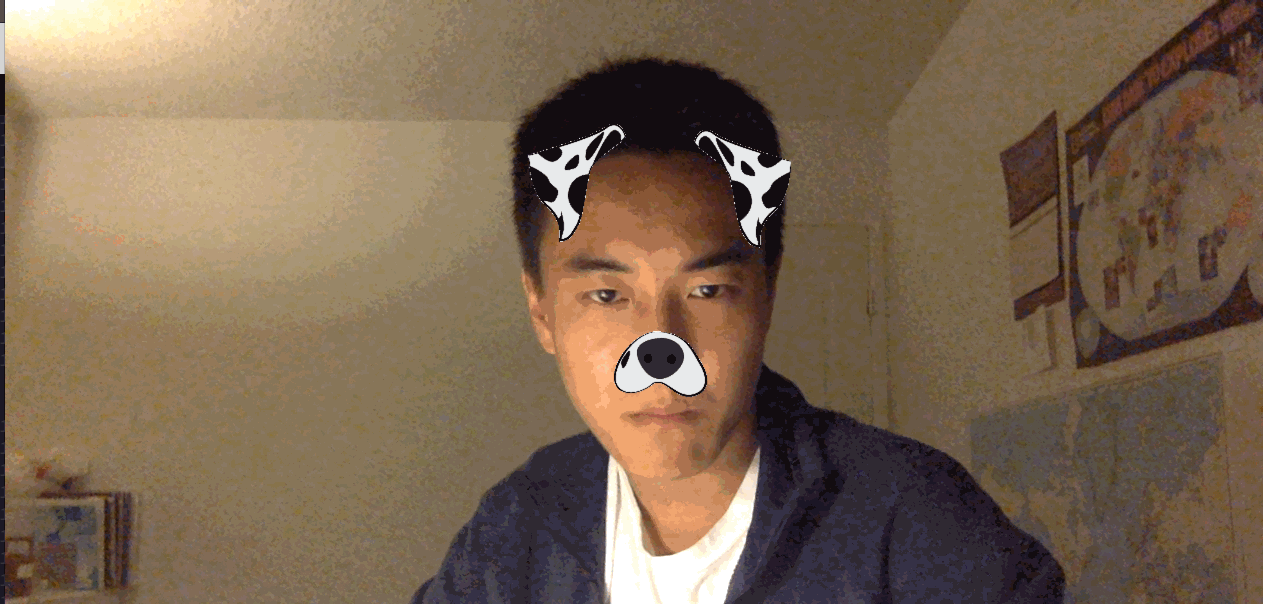This concludes our emotion-based dog filter and foray into computer vision.

## Conclusion

In this tutorial, you built a face detector and dog filter using computer vision and employed machine learning models to apply masks based on detected emotions.

Machine learning is widely applicable. However, it's up to the practitioner to consider the ethical implications of each application when applying machine learning. The application you built in this tutorial was a fun exercise, but remember that you relied on OpenCV and an existing dataset to identify faces, rather than supplying your own data to train the models. The data and models used have significant impacts on how a program works.

For example, imagine a job search engine where the models were trained with data about candidates. such as race, gender, age, culture, first language, or other factors. And perhaps the developers trained a model that enforces sparsity, which ends up reducing the feature space to a subspace where gender explains most of the variance. As a result, the model influences candidate job searches and even company selection processes based primarily on gender. Now consider more complex situations where the model is less interpretable and you don't know what a particular feature corresponds to. You can learn more about this in Equality of Opportunity in Machine Learning by Professor Moritz Hardt at UC Berkeley.

There can be an overwhelming magnitude of uncertainty in machine learning. To understand this randomness and complexity, you'll have to develop both mathematical intuitions and probabilistic thinking skills. As a practitioner, it is up to you to dig into the theoretical underpinnings of machine learning.

## OpenCV Python Tutorial: Computer Vision With OpenCV In Python

OpenCV Python Tutorial: Computer Vision With OpenCV In Python: Learn Vision Includes all OpenCV Image Processing Features with Simple Examples. Face Detection, Face Recognition. Computer Vision is an AI based, that is, Artificial Intelligence based technology that allows computers to understand and label images. Use OpenCV to work with image files. Create Face Detection Software. Detect Objects, including corner, edge, and grid detection techniques with OpenCV and Python. Use Python and Deep Learning to build image classifiers. Use Python and OpenCV to draw shapes on images and videos. Create Color Histograms with OpenCV

## OpenCV Python for Beginners - Learn Computer Vision with OpenCV 2020

OpenCV Python for Beginners - Learn Computer Vision with OpenCV in 10 Hours (2020). You'll learn: Introduction to OpenCV; How to Install OpenCV for Python on Windows 10; How to Read, Write, Show Images in OpenCV; How to Read, Write, Show Videos from Camera in OpenCV; matplotlib with OpenCV; Image Pyramids with Python and OpenCV; Canny Edge Detection in OpenCV; Image Blending using Pyramids in OpenCV; Face Detection using Haar Cascade Classifiers ...

## Machine Learning, Data Science and Deep Learning with Python

Complete hands-on Machine Learning tutorial with Data Science, Tensorflow, Artificial Intelligence, and Neural Networks. Introducing Tensorflow, Using Tensorflow, Introducing Keras, Using Keras, Convolutional Neural Networks (CNNs), Recurrent Neural Networks (RNNs), Learning Deep Learning, Machine Learning with Neural Networks, Deep Learning Tutorial with Python